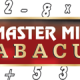## 05 Jul Mathematical Quest 1: Multiplication Tricks

The editor of the English section of Gonit Sora, writes a monthly column devoted to mathematics in a teen magazine called Young NE. The editor of Young NE was kind enough to grant us permission to republish the column after it appears on print. Below is the column that appeared in Vol. 1, Issue 1, May 2013 of Young NE.

First let us start from a lower set of numbers. Say you want to multiply 98 with 91. The usual technique would be to do it by hand in a paper and the answer would come out to be 8918. But that seems to be a waste of time really, when you can do it in a better and easier way. I have deliberately taken numbers nearer to 100, but the method will work for any number provided you know how to improvise quickly. I explain the steps in the algorithm below:

Step 1:

Identify the nearest multiple of 10 to both the numbers. Here the nearest to 98 is 100 and the nearest to 91 is 90. But for simplicity if there is a multiple of 100 nearby then we shall choose that number. So, for our purpose we take the number 100.

Step 2:

Subtract from the number obtained in Step 1, the numbers that are given to you for multiplication.

100-98=02

100-91=09

Step 3:

Now subtract the numbers obtained in Step 2 in the reverse order with the original numbers given. That is,

98-09=89

91-02=89

You will notice that you get the same number in this step. If however, you get two different numbers then you have done a mistake, and you should check your steps again.

Step 4:

Multiply the number obtained in the previous step with the multiple of 10 chosen in Step 1.

89 X 100=8900

Step 5:

Multiply the two numbers obtained in Step 2.

02 X 09=18

Step 6:

Add the numbers obtained in Step 4 and Step 5:

8900+18=8918

This may seem to be a long process, but once you get accustomed to it, then you will be surprised to see that you can do some really tough multiplication in your head.

The process can be made to work with any two numbers close to one another, like say 56 and 60, or 45 and 47. In these cases the appropriate multiple of 10 would be 50.

Now coming back to the three digit multiplication we mentioned at the beginning, I shall illustrate the steps below:

Step 1: The multiple to be chosen is 1000

Step 2: 1000-997=03, 1000-991=09

Step 3: 997-09=988, 991-03=988

Step 4: 988000

Step 5: 27

Step 6: 988027

And hence we obtained the answer!

A challenge for the students would be to mathematically justify using algebra why this method is correct. [Hint: You will have to use the formula for (a+b)(a+b).]

National Mathematics Year (2012):

The Government of India has declared 2012 as the National Mathematics Year as December 22nd, 2012 happens to be the 125th birth anniversary of the great Indian mathematician, S. Ramaujan. It is being celebrated with lots of activities all over India. An Assamese translation of Ramanujan’s biography is also underway and is hoped to be released on the occasion.

If you have any queries, comments, suggestions to make then please feel free to email at [email protected] or call/SMS at +91 80119 02141.

Download this post as PDF (will not include images and mathematical symbols).

##### Tags:
, , ,
•##### johnclark
Posted at 13:28h, 13 July Reply

Vedic maths is based on sixteen sutra’s or principles . These principles are general in nature and can be applied in many ways . In practice, the vedic system is used to solve difficult problems or huge sums in more effective and easy way.These method are just a part of a complete system of mathematics which is far more systematic than the modern system taught now. The simplicity of vedic mathematics helps us to solve the calculations mentally with sifficient practice.
Vedic maths is highly helpful in cracking competitive exams .There are many vedic math online course one of them is http://www.wiziq.com/course/1507-vedic-mathematics-complete-certified-course So if anybody is intrusted to learn vedic math online can visit the link.

•##### Gonit Sora
Posted at 12:04h, 16 July Reply

Thanks a lot for sharing this info with us.

•##### sid sahu
Posted at 17:15h, 31 August Reply

I am very much pleased with the contents you have mentioned. I enjoyed every little bit part of it.
It contains truly information. I want to thank you for this informative read; I really appreciate sharing this great.

————————–

•##### Gonit Sora
Posted at 23:22h, 04 September Reply

Thanks a lot, do keep visiting.

•##### Daynacook
Posted at 11:29h, 05 September Reply

Thanks for sharing up–to-date on this subject! I find it is very informative and very well written one! Keep up on this quality!
———-

•##### Gonit Sora
Posted at 12:55h, 05 September Reply

Thanks a lot, do keep visiting.